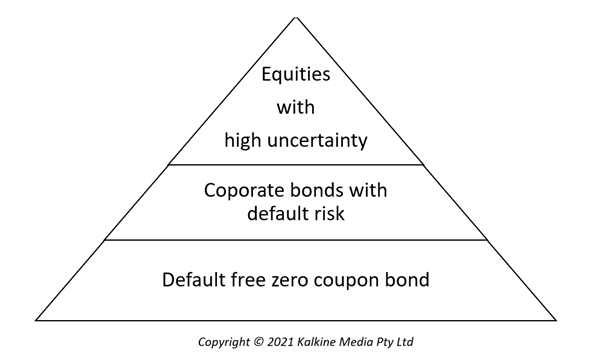# Discounted Cash Flow or DCF

• Updated on

Discounted Cash Flow is an investment valuation method estimating the present value of the future cash flows expected from the concerned investment. The discounted cash flow approach of valuation is the foundation on which all other valuation approaches are built like the relative valuation approach and contingent claim valuation approach. The DCF method may be used for valuation of a business, of shares, of investments, of projects or of bonds.

Summary
• DCF is a fundamental investment valuation method estimating the present value of the future cash flows expected from the concerned investment.
• The discounting rate factors in the riskiness of the financing mix.
• The equity valuation path or firm valuation path may be used for valuation purpose.

What is the foundation of DCF approach?

In the hierarchy the following is the nature of risk of the following assets:The least risky are the zero coupon bonds which yield regular cash flows and discounting these cash flows should give the value of the bond.  In case of corporate bonds which are riskier, the valuation is done by discounting the cash flows with an interest rate that takes into account the risk factor as well. Equities are the riskiest investments and their value is the present value of the future cash flows at a discount rate decided by weighing in the uncertainty too. Thus, we see that the underpinning of DCF method of valuation is to find the intrinsic value of the asset by attaching the right discount rate by assessing its fundamentals.

How is a business valued using the Discounted Cash Flow method?

A business can be valued either by valuing just the equity or equity, bonds, preferred stocks. The former may be called equity valuation and latter firm valuation.

In case of equity valuation, the present value is taken only on the claims on the firm’s equity while in the case of firm valuation present value is found on all the claims on the firm. Both approaches ideally would yield the same result if there is consistency in assumptions.

How is equity valuation path used to evaluate a business?Image Source: © Montrinipit | Megapixl.com

Equity valuation is arrived at by discounting the cash flows derived from the equities. Free cash flow to equity is the most widely used approach of equity valuation. Free cash flow to equity comprises the residual cash flows after expenses, capital expenditure needs, tax payments and debt servicing. Estimating this value may be a complex process.

From a common investor’s perspective cash flow from equity is the dividend thus it is a feasible proxy when individuals what to weigh in their investment options. This method is also called dividend discounted model. Here expected future dividends are discounted to arrive at the equity value.

Similarly, due to the ease of availability of data Earnings Per Share (EPS) may also be used as next best proxy especially in case of companies which are not regular on dividends.

Discounting rate used to discount the cash flows is the cost of equity as the numerator in the following formula is completely attributed to the equity holders-Where

CF to equityt = expected cash flow to equity in period t

k= cost of equity

n= life of the asset

How is Firm valuation path used to valuate a business?

Since the entire firm is being valued, here residual cash flows attributable to the business is discounted and this is free cash flow to firm. Free cash flow to firm comprises the residual cash flows after expenses, capital expenditure needs, tax payments but before debt servicing and payments to equity holders.

The Weighted Average Cost of Capital (WACC) is used for the purpose of discounting the cash flows. WACC is the average cost of financing used by the firm and may include a mix of debt, equity and a hybrid of the two (for example preferred shares)Where

CF to firmt = expected cash flow to firm in period t

WACC= Weighted Average Cost of Capital

n= life of the asset

How is WACC estimated?The cost of equity may be estimated using the Capital Asset Pricing Model (CAPM) while cost of debt should be estimated asBoth the paths of valuation- equity valuation and firm valuation must yield the same results provided that the underlying assumptions are used throughout.

What are the limitations of DCF formula discussed above?

The DCF method may not be useful for firms that are not performing well. Distressed firms may have negative cash flows, may not be a going concern and may face fear of bankruptcy. Similarly, companies undergoing restructuring process may make difficult the process of cash flow assessment.

Cash flow for Cyclical firms follow the crest and trough of the business cycle therefore to zero down on an assets cash flow is not a predictable task.

There may be unutilized assets which do not generate cash flows and the DCF method is not useful in this case. It may be a case of patents that are not in use currently but may have the possibility of future use.

In case a company is involved in acquisition, the synergies from the acquired entity must also be factored into the estimations.

Estimating the cost of equity for private firms may often be difficult due to a lack of history and thus there may be a reliance on peers which may be inefficient.

The bottom line of these drawbacks is not that DCF method cannot be used. It only means that the valuation process must be tweaked and made flexible to adapt to the situation.

##### Top ASX Listed Companies

Definition:
We use cookies to ensure that we give you the best experience on our website. If you continue to use this site we will assume that you are happy with it. OK